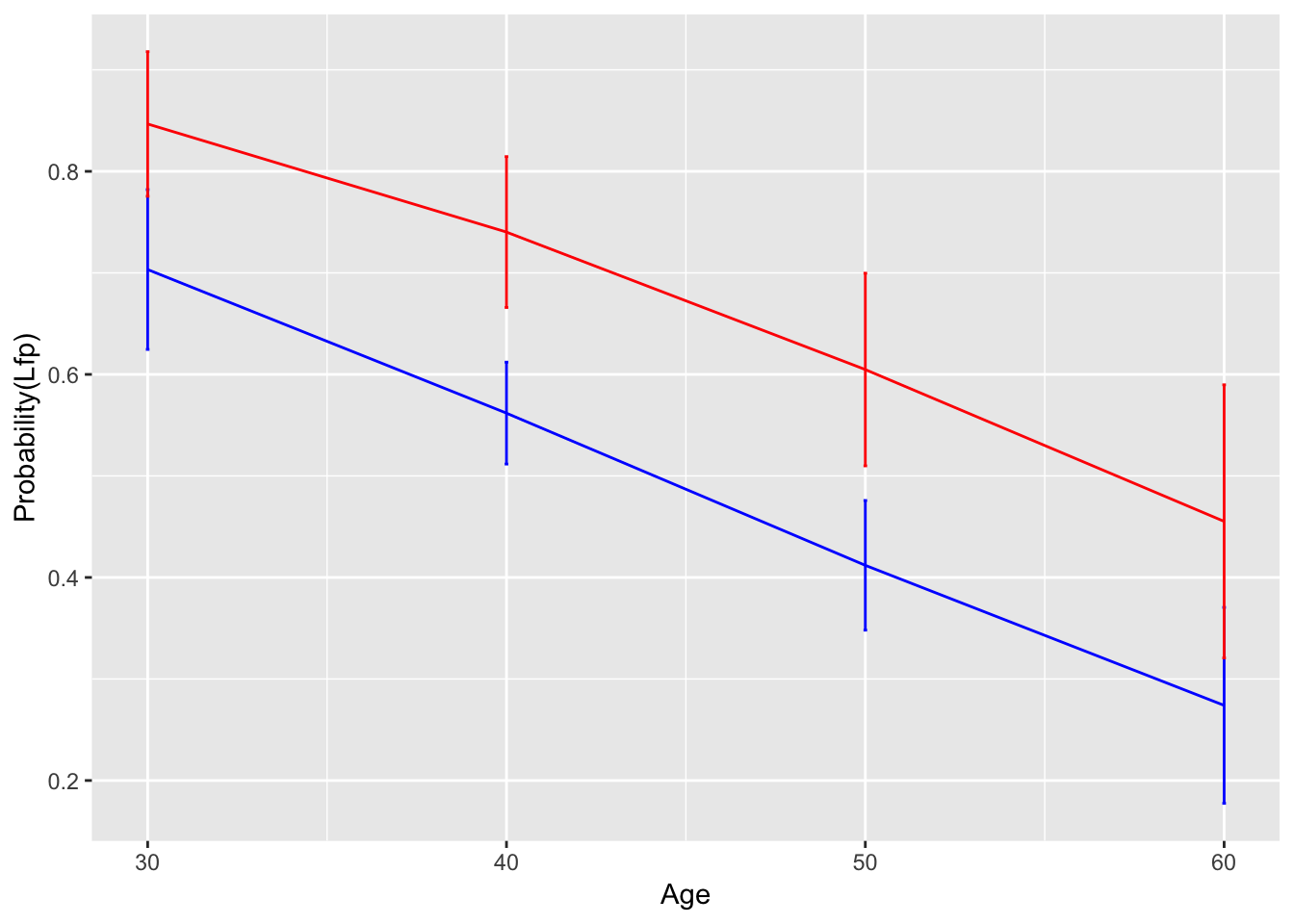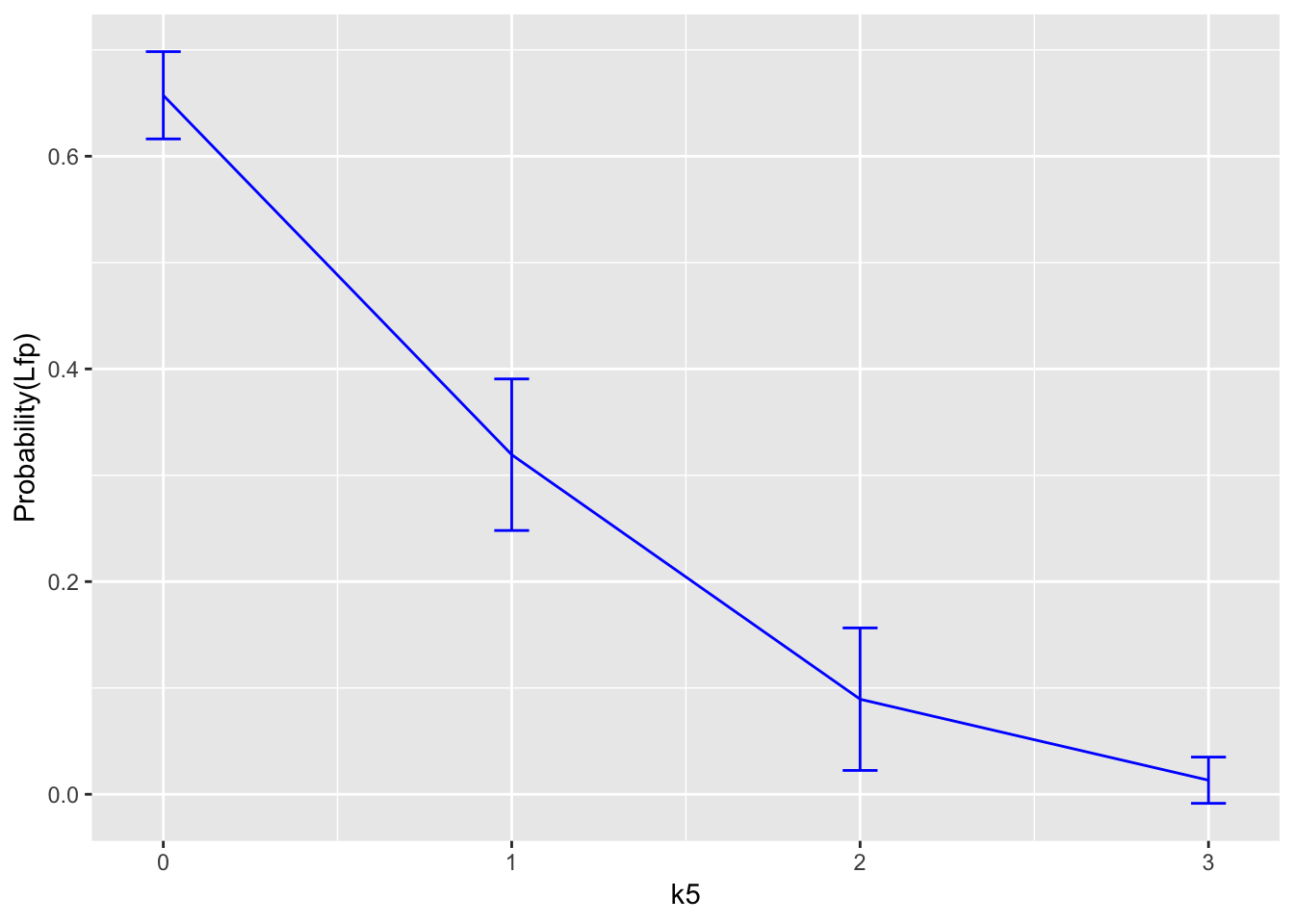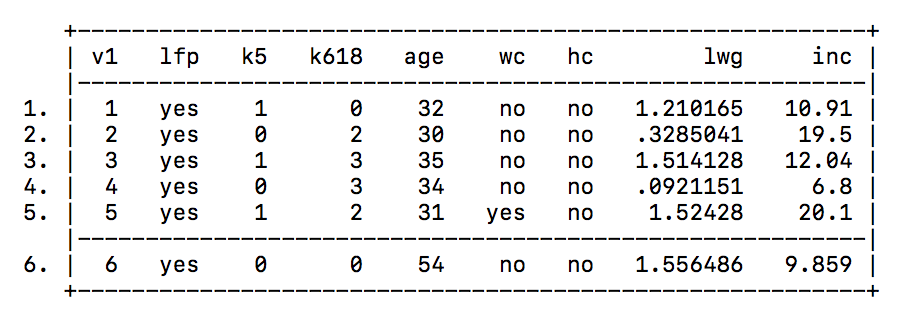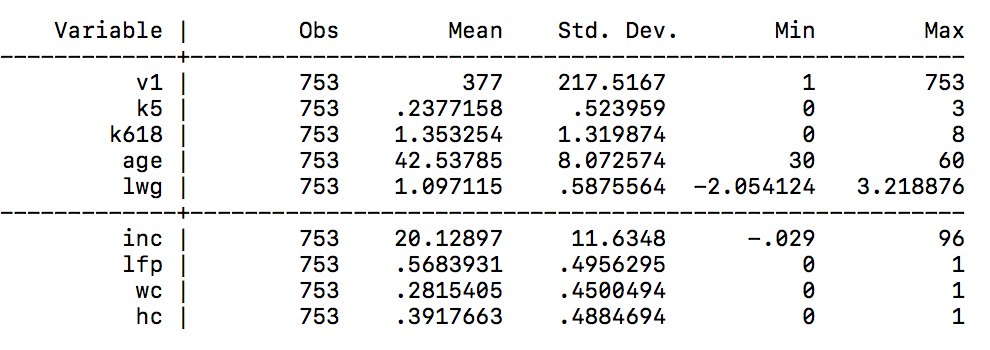The purpose of this tutorial is to provide a basic understanding of Probit Regression and its implementation in R, Python, Stata, and SAS, using the “Female Labor Force Participation” data set.

## Model Introduction

A probit regression is a version of the generalized linear model used to model dichotomous outcome variables. It uses the inverse standard normal distribution as a linear combination of the predictors. The binary outcome variable Y is assumed to have a Bernoulli distribution with parameter p (where the success probability is $$p \in (0,1)$$). Hence, the probit link function is $probit(EY) = \Phi^{-1}(p_i) = \Phi^{-1}(P_i[Y=1]) = \sum_{k=0}^{k=n}\beta_kx_{ik}$ where $\Phi=\frac{1}{\sqrt{2\pi}} \int_{-\infty}^{\alpha+\beta X} exp(-\frac{1}{2}Z^2)dZ$.

## Dataset: Female Labor Participation

In this tutorial, we work on Mroz data set on female labor participation with 8 variables. The data covers a sample of 753 married white women aged between 30 and 60 collected in 1975. The original source for this data is Mroz, T.A. (1987). “The sensitivity of an empirical model of married women’s hours of work to economic and statistical assumptions.” Econometrica 55, 765-799. The subset of the original data set used in this study can be found here. The description of the variables can be found below.

Variable(s) Description Type
lfp Labor-force participation of the married white woman Categorical: 0/1
k5 Number of children younger than 6 years old Positive integer
k618 Number of children aged 6-18 Positive integer
age Age in years Positive integer
wc Wifes college attendance Categorical: 0/1
hc Husbands college attendance Categorical: 0/1
lwg Log expected wage rate for women in the labor force Numerical
inc Family income without the wifes income Numerical

Our task is to identify whether certain characteristics of a woman’s household and personal life can predict her labor-force participation.

## Languages

### R

#### 1. Data Summary

First, we load data into R by using read.csv given that the data is in a comma-separated format.

mroz = read.csv('https://vincentarelbundock.github.io/Rdatasets/csv/carData/Mroz.csv')

This is what the first six rows look like in R:

head(mroz)
##   X lfp k5 k618 age  wc hc       lwg    inc
## 1 1 yes  1    0  32  no no 1.2101647 10.910
## 2 2 yes  0    2  30  no no 0.3285041 19.500
## 3 3 yes  1    3  35  no no 1.5141279 12.040
## 4 4 yes  0    3  34  no no 0.0921151  6.800
## 5 5 yes  1    2  31 yes no 1.5242802 20.100
## 6 6 yes  0    0  54  no no 1.5564855  9.859

Then, we change all binary variables to be numeric.

mroz$lfp = ifelse(mroz$lfp=="yes", 1, 0)
mroz$wc = ifelse(mroz$wc=="yes", 1, 0)
mroz$hc = ifelse(mroz$hc=="yes", 1, 0)

Here is the quick summary of the data.

summary(mroz)
##        X            lfp               k5              k618
##  Min.   :  1   Min.   :0.0000   Min.   :0.0000   Min.   :0.000
##  1st Qu.:189   1st Qu.:0.0000   1st Qu.:0.0000   1st Qu.:0.000
##  Median :377   Median :1.0000   Median :0.0000   Median :1.000
##  Mean   :377   Mean   :0.5684   Mean   :0.2377   Mean   :1.353
##  3rd Qu.:565   3rd Qu.:1.0000   3rd Qu.:0.0000   3rd Qu.:2.000
##  Max.   :753   Max.   :1.0000   Max.   :3.0000   Max.   :8.000
##       age              wc               hc              lwg
##  Min.   :30.00   Min.   :0.0000   Min.   :0.0000   Min.   :-2.0541
##  1st Qu.:36.00   1st Qu.:0.0000   1st Qu.:0.0000   1st Qu.: 0.8181
##  Median :43.00   Median :0.0000   Median :0.0000   Median : 1.0684
##  Mean   :42.54   Mean   :0.2815   Mean   :0.3918   Mean   : 1.0971
##  3rd Qu.:49.00   3rd Qu.:1.0000   3rd Qu.:1.0000   3rd Qu.: 1.3997
##  Max.   :60.00   Max.   :1.0000   Max.   :1.0000   Max.   : 3.2189
##       inc
##  Min.   :-0.029
##  1st Qu.:13.025
##  Median :17.700
##  Mean   :20.129
##  3rd Qu.:24.466
##  Max.   :96.000

#### 2. Fitting model by Probit Regression

Next, we run a probit regression using lfp as a response variable and all the remaining variables as predictors.

mroz.probit <- glm(lfp ~ k5 + k618 + age + wc + hc + lwg + inc,
data = mroz,
family = binomial(link = "probit"))
summary(mroz.probit)
##
## Call:
## glm(formula = lfp ~ k5 + k618 + age + wc + hc + lwg + inc, family = binomial(link = "probit"),
##     data = mroz)
##
## Deviance Residuals:
##     Min       1Q   Median       3Q      Max
## -2.1359  -1.1024   0.5967   0.9746   2.2236
##
## Coefficients:
##              Estimate Std. Error z value Pr(>|z|)
## (Intercept)  1.918418   0.382356   5.017 5.24e-07 ***
## k5          -0.874712   0.114423  -7.645 2.10e-14 ***
## k618        -0.038595   0.040950  -0.942 0.345942
## age         -0.037824   0.007605  -4.973 6.58e-07 ***
## wc           0.488310   0.136731   3.571 0.000355 ***
## hc           0.057172   0.124207   0.460 0.645306
## lwg          0.365635   0.089992   4.063 4.85e-05 ***
## inc         -0.020525   0.004852  -4.230 2.34e-05 ***
## ---
## Signif. codes:  0 '***' 0.001 '**' 0.01 '*' 0.05 '.' 0.1 ' ' 1
##
## (Dispersion parameter for binomial family taken to be 1)
##
##     Null deviance: 1029.75  on 752  degrees of freedom
## Residual deviance:  905.39  on 745  degrees of freedom
## AIC: 921.39
##
## Number of Fisher Scoring iterations: 4

All of the coefficients, except for k618 and hc, are statistically significant.

We can also obtain confidence intervals for the coefficient estimates.

confint(mroz.probit)
##                   2.5 %      97.5 %
## (Intercept)  1.17740788  2.66999634
## k5          -1.10050075 -0.65525817
## k618        -0.11806899  0.04069610
## age         -0.05283742 -0.02300114
## wc           0.22421495  0.75547759
## hc          -0.18586158  0.30035566
## lwg          0.19375227  0.53805204
## inc         -0.02999733 -0.01126605

#### 3. Marginal effect

We first make a cross-tab of categorical predictors with our binary response variable and calculate adjusted predictions of lfp for two levels of hc and wc variables.

##### Group by hc

We start by tabulating lfp and hc.

addmargins(table(mroz$lfp, mroz$hc, deparse.level=2))
##         mroz$hc ## mroz$lfp   0   1 Sum
##      0   207 118 325
##      1   251 177 428
##      Sum 458 295 753

Then, we calculate the adjusted predictions of lfp for the two levels of hc variable.

hc_data0 = data.frame(k5 = mean(mroz$k5), k618 = mean(mroz$k618), age=mean(mroz$age), lwg= mean(mroz$lwg), inc=mean(mroz$inc), hc=0, wc=mean(mroz$wc))
hc_data1 = data.frame(k5 = mean(mroz$k5), k618 = mean(mroz$k618), age=mean(mroz$age), lwg= mean(mroz$lwg), inc=mean(mroz$inc), hc=1, wc=mean(mroz$wc))
h0 = predict(mroz.probit, hc_data0, type="response", se=TRUE)
h1 = predict(mroz.probit, hc_data1, type="response", se=TRUE)
hc_fit = data.frame(Margin = c(h0$fit, h1$fit), se=c(h0$se.fit, h1$se.fit))
hc_fit
##      Margin         se
## 1 0.5693807 0.02726260
## 2 0.5917191 0.03463592

The marginal probability of husband being a college graduate is is 0.59, while the marginal probability of husband being a high school graduate is slightly lower at 0.57.

##### Group by Wife’s College Attendance

Here is the tabulation of lfp and wc. As can be seen below, there is a significant imbalance between labor force participation, when the women are college graduates.

addmargins(table(mroz$lfp, mroz$wc, deparse.level=2))
##         mroz$wc ## mroz$lfp   0   1 Sum
##      0   257  68 325
##      1   284 144 428
##      Sum 541 212 753

We also calculate the adjusted predictions of lfp for the two levels of wc variable, while keeping other variables at mean.

wc_data0 = data.frame(k5 = mean(mroz$k5), k618 = mean(mroz$k618), age=mean(mroz$age), lwg= mean(mroz$lwg), inc=mean(mroz$inc), hc = mean(mroz$hc), wc=0)
wc_data1 = data.frame(k5 = mean(mroz$k5), k618 = mean(mroz$k618), age=mean(mroz$age), lwg= mean(mroz$lwg), inc=mean(mroz$inc), hc = mean(mroz$hc), wc=1)
w0 = predict(mroz.probit, wc_data0, type="response", se=TRUE)
w1 = predict(mroz.probit, wc_data1, type="response", se=TRUE)
wc_fit = data.frame(Margin = c(w0$fit, w1$fit), se=c(w0$se.fit, w1$se.fit))
wc_fit
##      Margin         se
## 1 0.5238094 0.02402095
## 2 0.7081631 0.03847631

The marginal probability increases from 0.524 to 0.708 when women are college graduates vs. only high-school graduates.

##### Group by Age and Wife’s College Attendance

We can predict the outcome variable by both age and women’s college education. We will use increments of 10 between 30 and 60 as representative ages. As the results show, the older a woman gets, the less are her chances of participating in the labor force. Regardless of age, women’s college education always yields a higher probability of labor force participation.

wc_data0_age=data.frame(k5=rep(mean(mroz$k5), 4), k618=rep(mean(mroz$k618), 4),
age=c(30, 40, 50, 60), lwg=rep(mean(mroz$lwg), 4), inc=rep(mean(mroz$inc), 4), wc=rep(0, 4),
hc=rep(mean(mroz$hc), 4)) wc_data1_age=data.frame(k5=rep(mean(mroz$k5), 4), k618=rep(mean(mroz$k618), 4), age=c(30, 40, 50, 60), lwg=rep(mean(mroz$lwg), 4),
inc=rep(mean(mroz$inc), 4), wc=rep(1, 4), hc=rep(mean(mroz$hc), 4))
m0=predict(mroz.probit, wc_data0_age, type="response", se=TRUE)
m1=predict(mroz.probit, wc_data1_age, type="response", se=TRUE)
wc_fit_age = data.frame(Age=c(30, 40, 50, 60),Margin_wc0=m0$fit, Margin_wc1=m1$fit, se_wc0=m0$se.fit, se_wc1=m1$se.fit)
wc_fit_age
##   Age Margin_wc0 Margin_wc1     se_wc0     se_wc1
## 1  30  0.7033092  0.8466691 0.03936089 0.03561642
## 2  40  0.5618680  0.7402177 0.02509639 0.03716213
## 3  50  0.4119514  0.6047963 0.03193009 0.04744612
## 4  60  0.2739989  0.4552319 0.04823869 0.06726396

Using ggplot, we can plot the marginal effects to visualize the output. As can be seen from the plot, the variability of predictions increase with the age. Nevertheless, it is clear that a woman’s labor force participation is strongly affected by her age.

ggplot(data=wc_fit_age,aes(y=Age)) +
geom_line(aes( x= Margin_wc0), colour="blue") +
geom_errorbarh(aes(xmin=Margin_wc0-2*se_wc0, xmax=Margin_wc0+2*se_wc0), height=.1, colour="blue")+
geom_line(aes(x = Margin_wc1), colour="red")+
geom_errorbarh(aes(xmin=Margin_wc1-2*se_wc1, xmax=Margin_wc1+2*se_wc1),
height=.1,colour="red")+scale_color_manual(labels = c("wc=0","wc=1"), values = c("blue", "red"))+
coord_flip()+xlab('Probability(Lfp)') +
ylab("Age")##### Group by Number of Children 5 years or younger

Below is a cross-tab of lfp with k5. The tabulation shows that the more the number of kids aged below 6 years, the less women participate in the labor force.

addmargins(table(mroz$lfp, mroz$k5, deparse.level=2))
##         mroz$k5 ## mroz$lfp   0   1   2   3 Sum
##      0   231  72  19   3 325
##      1   375  46   7   0 428
##      Sum 606 118  26   3 753

We can predict lfp at different levels of k5, by keeping other variables at mean. When k5=0, the marginal probability of a woman’s participation in the labor force is 0.657, whereas when they have three or more, the probability is at around 0.013. This range is a good indicator of the significance of k5 variable.

k_data=data.frame(k5=c(0,1,2,3),
k618=rep(mean(mroz$k618), 4), age=rep(mean(mroz$age), 4),
lwg=rep(mean(mroz$lwg), 4), inc=rep(mean(mroz$inc), 4),
wc = rep(mean(mroz$wc), 4), hc=rep(mean(mroz$hc), 4))
k0=predict(mroz.probit, k_data, type="response", se=TRUE)
k_fit = data.frame(k5=c(0,1,2,3),Margin_k=k0$fit, se_k=k0$se.fit)
k_fit
##   k5   Margin_k       se_k
## 1  0 0.65730850 0.02052209
## 2  1 0.31932620 0.03563207
## 3  2 0.08942632 0.03349808
## 4  3 0.01324307 0.01087202

We can also plot the marginal effects of k5. As the plot below shows, there is a significant drop in the probability of a woman being employed with the increase in the number of children below the age of 6.

ggplot(data=k_fit,aes(y=k5)) +
geom_line(aes( x= Margin_k), colour="blue") +
geom_errorbarh(aes(xmin=Margin_k-2*se_k, xmax=Margin_k+2*se_k), height=.1, colour="blue")+
coord_flip()+xlab('Probability(Lfp)') +
ylab("k5")### Python

#### 1. Data Summary

First we start by loading the data into memory using the “pandas” package. The data is read in as a pandas dataframe, which is very similar to a R dataframe.

We also load “numpy” for array manipulation and we will be using the “statsmodel” package for Probit regression.

import pandas as pd
import numpy as np
from statsmodels.discrete.discrete_model import Probit
data = pd.read_csv('https://vincentarelbundock.github.io/Rdatasets/csv/carData/Mroz.csv')

To print the first few rows of the data, we use the head function. This is similar to other languages such as R.

print(data.head())
   Unnamed: 0  lfp  k5  k618  age   wc  hc       lwg        inc
0           1  yes   1     0   32   no  no  1.210165  10.910001
1           2  yes   0     2   30   no  no  0.328504  19.500000
2           3  yes   1     3   35   no  no  1.514128  12.039999
3           4  yes   0     3   34   no  no  0.092115   6.800000
4           5  yes   1     2   31  yes  no  1.524280  20.100000

#### Data Cleaning

Since some of the data is read in as strings, we need to transform them into binary categorical data using the following code. We also drop the first column as it is read in with row numbers, which we do not need.

data = data.drop(data.columns, axis = 1)
data["lfp"] = data["lfp"] == "yes"
data["wc"] = data["wc"] == "yes"
data["hc"] = data["hc"] == "yes"

Looking at the data again we see:

    lfp  k5  k618  age     wc     hc       lwg        inc
0  True   1     0   32  False  False  1.210165  10.910001
1  True   0     2   30  False  False  0.328504  19.500000
2  True   1     3   35  False  False  1.514128  12.039999
3  True   0     3   34  False  False  0.092115   6.800000
4  True   1     2   31   True  False  1.524280  20.100000

#### Summary Statistics

Before we begin our analysis, here is a quick summary of the data. We use the function “describe” on our dataframe to generate some summary statistics.

print(data.describe())

This generates summary statistics for the continuous variables in our dataset.

               k5        k618         age         lwg         inc
count  753.000000  753.000000  753.000000  753.000000  753.000000
mean     0.237716    1.353254   42.537849    1.097115   20.128965
std      0.523959    1.319874    8.072574    0.587556   11.634799
min      0.000000    0.000000   30.000000   -2.054124   -0.029000
25%      0.000000    0.000000   36.000000    0.818086   13.025000
50%      0.000000    1.000000   43.000000    1.068403   17.700001
75%      0.000000    2.000000   49.000000    1.399717   24.466000
max      3.000000    8.000000   60.000000    3.218876   96.000000

#### 2. Fitting Probit Regression

First we break our dataset into response variable and predictor variables. We will use lfp as our response variable and all the remaining variable as predictors. Then we use the statsmodels function to fit our Probit regression with our response variable and design matrix. The statsmodels package is unique from other languages and packages as it does not include an intercept term by default. This needs to be manually set.

Y = data["lfp"]
X = data.drop(["lfp"], 1)
model = Probit(Y, X.astype(float))
probit_model = model.fit()
print(probit_model.summary())

The following is the results of our regression. The statsmodels package automatically includes p values and confidence intervals for each coefficient. For those that are familiar with objects, the probit model is stored as a probit model object in Python. All operations with the model are invoked as model.member_function().

Optimization terminated successfully.
Current function value: 0.601189
Iterations 5
Probit Regression Results
==============================================================================
Dep. Variable:                    lfp   No. Observations:                  753
Model:                         Probit   Df Residuals:                      745
Method:                           MLE   Df Model:                            7
Date:                Fri, 07 Dec 2018   Pseudo R-squ.:                  0.1208
Time:                        01:40:27   Log-Likelihood:                -452.69
converged:                       True   LL-Null:                       -514.87
LLR p-value:                 9.471e-24
==============================================================================
coef    std err          z      P>|z|      [0.025      0.975]
------------------------------------------------------------------------------
const          1.9184      0.381      5.040      0.000       1.172       2.664
k5            -0.8747      0.114     -7.703      0.000      -1.097      -0.652
k618          -0.0386      0.040     -0.953      0.340      -0.118       0.041
age           -0.0378      0.008     -4.971      0.000      -0.053      -0.023
wc             0.4883      0.135      3.604      0.000       0.223       0.754
hc             0.0572      0.124      0.461      0.645      -0.186       0.300
lwg            0.3656      0.088      4.165      0.000       0.194       0.538
inc           -0.0205      0.005     -4.297      0.000      -0.030      -0.011
==============================================================================

It appears that all our predictors are statistically significant at level a except hc and k618.

#### 3. Marginal Effects

To better understand why our predictors are significant, we attempt to study the marginal effects of our predictors. In this section, we will look at the predictors hc, wc, age, and k5. Unfortunately, there is no member function of the probit class to calculate the standard error of each prediction so this is ommited in this version.

##### Group by Husband’s College Attendance

First we group the data by hc.

To better understand hc, we tabulate lfp and hc. This is done with the pandas crosstab function.

print(pd.crosstab(data["lfp"], data["hc"], margins = True))
hc     False  True  All
lfp
False    207   118  325
True     251   177  428
All      458   295  753

Then we calculate adjusted predictions of lfp for two levels of the hc variable. Creating these adjusted predictors is not very clean in Python compared to other languages. We have to build the array from scratch.

hc_data0 = np.column_stack((
1,
np.mean(data["k5"]),
np.mean(data["k618"]),
np.mean(data["age"]),
np.mean(data["wc"]),
0,
np.mean(data["lwg"]),
np.mean(data["inc"])
))

hc_data1 = np.column_stack((
1,
np.mean(data["k5"]),
np.mean(data["k618"]),
np.mean(data["age"]),
np.mean(data["wc"]),
1,
np.mean(data["lwg"]),
np.mean(data["inc"])
))

The results of running this prediction is as follows.

print(probit_model.predict(hc_data0))
print(probit_model.predict(hc_data1))
[ 0.56938181]
[ 0.59171968]

We see that the marginal probability of husband being a collage graduate is 0.59, while the marginal probability of husband being a high school graduate is lower at 0.57.

##### Group by Wife’s College Attendance

Similarly to hc, we tabulate our response variable lfp and predictor wc. We can see that there is a significant imbalance between labor force participation for women that are college graduates.

print(pd.crosstab(data["lfp"], data["wc"], margins = True))
wc     False  True  All
lfp
False    257    68  325
True     284   144  428
All      541   212  753

We also calculate the adjusted predictions of lfp for the two levels of wc variable, while keeping other variables at the mean.

wc_data0 = np.column_stack((
1,
np.mean(data["k5"]),
np.mean(data["k618"]),
np.mean(data["age"]),
0,
np.mean(data["hc"]),
np.mean(data["lwg"]),
np.mean(data["inc"])
))
wc_data1 = np.column_stack((
1,
np.mean(data["k5"]),
np.mean(data["k618"]),
np.mean(data["age"]),
1,
np.mean(data["hc"]),
np.mean(data["lwg"]),
np.mean(data["inc"])
))

The result of running this prediction is as follows:

print(probit_model.predict(wc_data0))
print(probit_model.predict(wc_data1))
[ 0.52380974]
[ 0.70816505]

It appears that the marginal probability increases from 0.524 to 0.708 when women are college graduates compared to highschool graduates.

##### Group by Age and Wife’s College Attendance

We can predict the outcome variable by both age and women’s college education. We use increments of 10 between 30 and 60 as our representative ages.

wc_data0 = np.column_stack((
np.repeat(1,4),
np.repeat(np.mean(data["k5"]),4),
np.repeat(np.mean(data["k618"]), 4),
(30,40,50,60),
np.repeat(0,4),
np.repeat(np.mean(data["hc"]),4),
np.repeat(np.mean(data["lwg"]),4),
np.repeat(np.mean(data["inc"]),4)
))
wc_data1 = np.column_stack((
np.repeat(1,4),
np.repeat(np.mean(data["k5"]),4),
np.repeat(np.mean(data["k618"]), 4),
(30,40,50,60),
np.repeat(1,4),
np.repeat(np.mean(data["hc"]),4),
np.repeat(np.mean(data["lwg"]),4),
np.repeat(np.mean(data["inc"]),4)
))

The results of running the prediction is as follows:

print(probit_model.predict(wc_data0))
print(probit_model.predict(wc_data1))
[ 0.70330952  0.5618684   0.41195181  0.27399923]
[ 0.84667045  0.74021954  0.6047985   0.45523423]

As we can see, the older a women gets, the less chance of her participating in the labor force. Regardless of age, women’s college education always yields a higher probability of labor force participation.

##### Group by Number of Children 5 years or younger (K5)

Finally we look at the effects of number of children 5 years or younger. As before, here is a cross tabulation of this variable.

print(pd.crosstab(data["lfp"], data["k5"], margins = True))
k5       0    1   2  3  All
lfp
False  231   72  19  3  325
True   375   46   7  0  428
All    606  118  26  3  753

Then we predict lfp at different levels of k5, while keeping other variables at their means.

k5_data = np.column_stack((
np.repeat(1,4),
(0,1,2,3),
np.repeat(np.mean(data["k618"]), 4),
np.repeat(np.mean(data["age"]),4),
np.repeat(np.mean(data["wc"]),4),
np.repeat(np.mean(data["hc"]),4),
np.repeat(np.mean(data["lwg"]),4),
np.repeat(np.mean(data["inc"]),4)
))

print(probit_model.predict(k5_data))
[ 0.65730924  0.31932735  0.08942703  0.01324326]

We see that if there are three or more children under the age of 5, there is a 0.013 chance of a woman being in the work force, but when there is no children there is a 0.6573 chance. This is a good indication of the significance of this variable.

##### Extension

Finally, an overall marginal effect can be observed by calling the get_margeff() method of the probit model class. This is unique to Python. Results are as follows.

mfx = probit_model.get_margeff()
print(mfx.summary())
 Probit Marginal Effects

=====================================
Dep. Variable:                    lfp
Method:                          dydx
At:                           overall
==============================================================================
dy/dx    std err          z      P>|z|      [0.025      0.975]
------------------------------------------------------------------------------
k5            -0.2997      0.034     -8.726      0.000      -0.367      -0.232
k618          -0.0132      0.014     -0.955      0.340      -0.040       0.014
age           -0.0130      0.002     -5.219      0.000      -0.018      -0.008
wc             0.1673      0.045      3.696      0.000       0.079       0.256
hc             0.0196      0.042      0.461      0.645      -0.064       0.103
lwg            0.1253      0.029      4.311      0.000       0.068       0.182
inc           -0.0070      0.002     -4.451      0.000      -0.010      -0.004
==============================================================================

### Stata

#### 1.Data Summary

Firstly, we import the Mroz data from the website and show the first six rows of the dataset.

*Importing data
import delimited https://vincentarelbundock.github.io/Rdatasets/csv/carData/Mroz.csv, clear
save mroz,replace
use mroz,clear
*List the first six rows
list if v1<=6Then, we change all binary variables to be numeric, and we get a summary of the data. Our response is lfp and its mean is 0.57. The range of age is from 30 to 60.

*Change variables with values yes/no to 1/0
gen lfpart =1 if lfp == "yes"
replace lfpart =0 if lfp == "no"
gen wifec =1 if wc == "yes"
replace wifec =0 if wc == "no"
gen husbc =1 if hc == "yes"
replace husbc =0 if hc == "no"
drop lfp wc hc
rename lfpart lfp
rename wifec wc
rename husbc hc
*Get the summary of the data
summ#### 2.Fitting model by Probit Regression

Now, we fit our data by probit regression. lfp is the response and the remaining variables are predictors. Looking at the p-values, all variables have high sigificance, except k618 and hc.

*Fitting the data by probit regression
probit lfp k5 k618 age lwg inc i.wc i.hc`